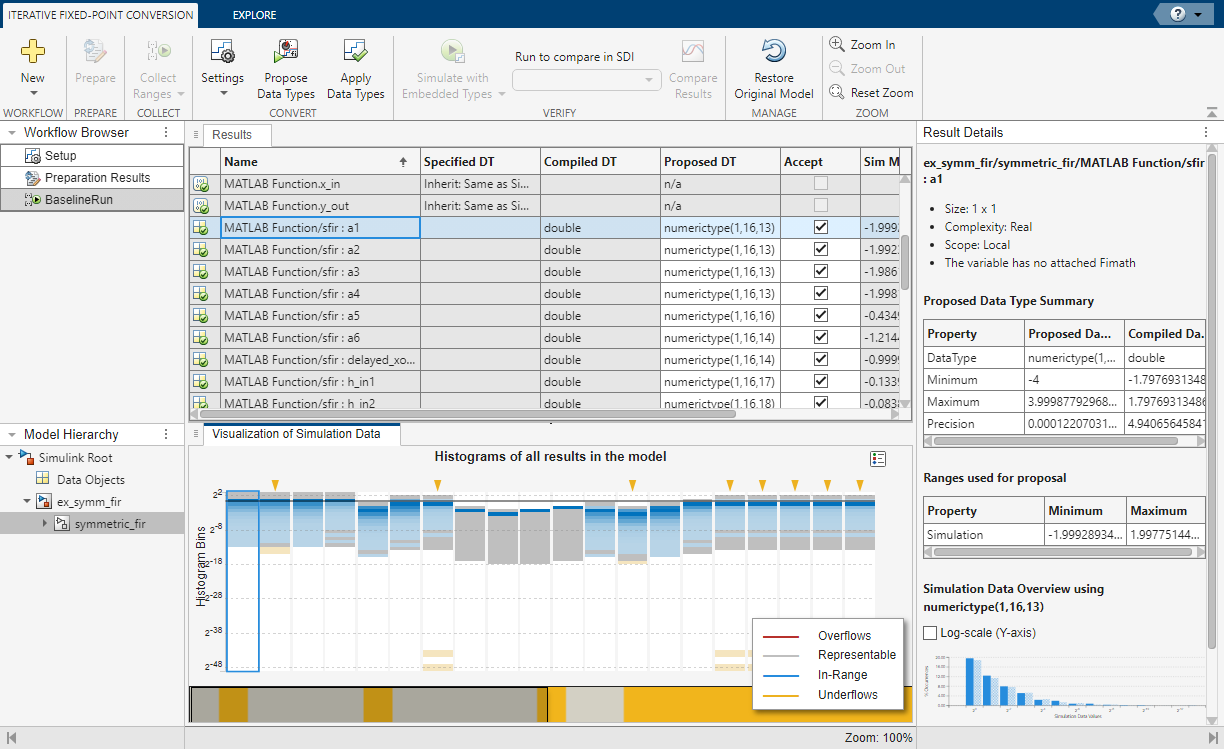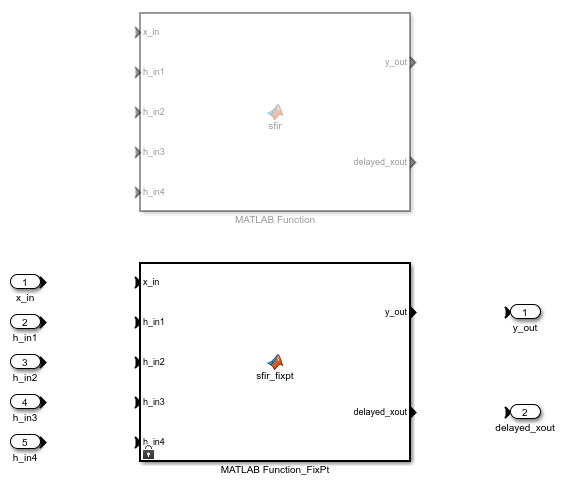## Convert MATLAB Function Block to Fixed Point

This example shows how to use the Fixed-Point Tool to convert a model containing a MATLAB® Function block to fixed point.

### Best Practices for Working with the MATLAB Function Block in the Fixed-Point Tool

• Do not edit the fixed-point variant of your MATLAB Function block algorithm. Use the code view to edit the floating-point variant of your MATLAB code and repropose and apply data types.

• For a successful conversion, only use modeling constructs supported by the Fixed-Point Converter app. For a list of the supported modeling constructs, see MATLAB Language Features Supported for Automated Fixed-Point Conversion.

• While collecting range information, do not edit the MATLAB code in the MATLAB Function block. Editing the code will cause problems if you try to merge results.

• During the fixed-point conversion process using the Fixed-Point Tool, do not use the “Save as” option to save the MATLAB Function block with a different name. If you do, you might lose existing results for the original block.

### Open the Model

Change directories to the folder where the model is located. At the MATLAB command line, enter:

```cd(fullfile(docroot,'toolbox','fixpoint','examples')) ```

Copy the `ex_symmetric_fir.slx` file to a local writable folder and open the model.The `ex_symmetric_fir` model uses a symmetric FIR filter. Simulate the model and inspect the model output. Inspect the symmetric FIR filter algorithm by double-clicking the MATLAB Function block.

1. To open the Fixed-Point Tool, in the Apps tab, expand the Apps gallery and select Fixed-Point Tool.

2. In the Fixed-Point Tool, under System Under Design (SUD), select the `symmetric_fir` subsystem, which contains the MATLAB Function block, as the system to convert.

3. Under Range Collection Mode, select Simulation ranges as the method of range collection. This configures the model to collect ranges using idealized floating-point data types.

4. In the Prepare System section of the toolstrip, click Prepare.

### Collect Range Information

Collect idealized ranges to use for data type proposal. Click the button to start the simulation.

The Fixed-Point Tool stores the simulation data in a run titled `Ranges(Double)`. Examine the range information of the MATLAB variables in the spreadsheet.

### Propose Data Types

Configure the proposal settings and propose fixed-point data types for the model.

1. In the Convert Data Types section of the toolstrip you can configure the data type proposal settings for the MATLAB Function block variables.

In this example, use the default proposal settings.

2. Click Propose Data Types.

The data type proposals appear in the ProposedDT column of the spreadsheet.

### Note

The SpecifiedDT column is always blank for MATLAB Function block variables.### Inspect Code Using the Code View

To launch the code view, click the MATLAB Functions button.

Using the code view you can:

To view the current proposal settings, click . Here you can edit the `fimath` properties for the function. For this example, the default `fimath` properties are sufficient.### Apply Proposed Data Types

When you have finished examining the proposed types, editing proposal settings, and implementing any function replacements, apply the proposed data types to the model. You can apply the data types either from the code view, or from the Fixed-Point Tool.

In the code view window, click . The left pane displays both the original floating-point MATLAB Function block, as well as a newly generated fixed-point variant MATLAB Function block.

Right-click on the MATLAB Function block node in the left pane. Select ```Go to Block``` to navigate to the MATLAB Function block in the model.A variant subsystem is now in the place of the MATLAB Function block. The variant subsystem contains both floating-point and fixed-point versions of the MATLAB Function block. The active version is automatically controlled by the Fixed-Point Tool based on the data type override settings of the model. Data Type Override is not currently active on the model, so the fixed-point version is active.### Verify Results

Return to the Fixed-Point Tool to verify the results of the conversion.

In the Verify section of the toolstrip, click the Simulate with Embedded Types button to simulate the model using the newly applied fixed-point data types. The model simulates with the fixed-point variant as the active variant.## Related Topics

##### SupportGet trial now